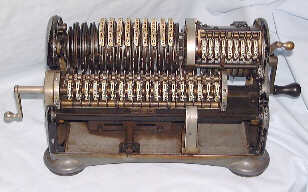# Mechanical MultiplicationBefore multiplication and division can be explained, it is necessary to know a little more about the calculator. Firstly, the figure wheels of the output register and their associated transfer gears and carry cams are mounted on a movable carriage which can be shifted to the left or to the right. In the illustration it has been shifted six places to the left. There is a movable pointer just below register two which indicates which column of figures is active (in this case, column six).

Secondly, register two, on the upper right of the machine, will increment the active column (and if necessary carry to the left) each time the crank handle completes one rotation.

Having got that over with, we can proceed and think about multiplying 456 by 123.

One way of doing this is to enter 456 on the setting levers and then turn the handle one hundred and twenty three times. This is the sort of thing that people used to do when they got bored but there is an easier way.

1. Shift the carriage as far LEFT as it will go
2. Set up 456 on the setting levers
3. Rotate the crank three times. This multiplies 456 by three, the registers show:-
```                              456     000000003
^
000000000000001368
```
4. Shift the carriage once to the right
5. Rotate the crank twice. This multiplies 456 by 20 and adds the result into the output register. The registers now show:-
```                              456     000000023
^
000000000000010488
```
6. Shift the carriage once to the right.
7. Rotate the crank handle once. This multiplies 456 by 100 and adds in the result. The registers now show:-
```                              456     000000123
^
000000000000056088
```
8. The calculation is complete.

## Mechanical Division

Division is accomplished by repeated subtractions, but the nice thing about division is that there is a little bell that rings every so often during the calculation.

We are going to divide 456 by 123

1. Shift the carriage as far to the RIGHT as it will go
2. Set up 456 on the setting levers
3. Rotate the crank once FORWARDS to transfer this to the output register - register 2 is incremented - the registers show:-
```                              456     100000000
^
000000045600000000
```
4. Clear the setting levers and register 2
```                              000     000000000
^
000000045600000000
```
5. Set up 123 on the setting levers
6. Set the multiply/divide lever to DIVIDE - this makes register 2 increment when the crank is turned backwards - some calculators will take care of this automatically.
7. Rotate the crank once BACKWARDS
```                              123     100000000
^
000000033300000000
```
8. Rotate the crank once backwards
```                              123     200000000
^
000000021000000000
```
9. Rotate the crank once backwards
```                              123     300000000
^
000000008700000000
```
10. Rotate the crank once backwards
```                              123     400000000
^
999999996400000000       (ping!!) - Warning -
we have gone too far
```
11. Rotate the crank once FORWARDS
```                              123     300000000
^
000000008700000000
```
12. Shift the carriage one place LEFT
```                              123     300000000
^
000000008700000000
```
13. Rotate the crank once BACKWARDS
```                              123     310000000
^
000000007470000000
```
14. Rotate the crank BACKWARDS until the nines come up again and the bell rings
```                              123     380000000
^
999999998860000000        (ping!!)
```
15. Rotate the crank FORWARD - we'll get it right next time
```                              123     370000000
^
000000000090000000
```
16. Shift the carriage one place LEFT
```                              123     370000000
^
000000000090000000
```
17. 123 is bigger than 90 so we need to shift again ...
18. Shift the carriage one place LEFT
```                              123     370000000
^
000000000090000000
```
19. Now, rotate the crank BACKWARDS until JUST BEFORE all the nines come up
```                              123     370700000
^
000000000003900000
```
20. Shift the carriage one place LEFT
```                              123     370700000
^
000000000003900000
```
21. Rotate the crank BACKWARDS until JUST BEFORE all the nines come up
```                              123     370730000
^
000000000000210000
```

And so on....

The result is 3.7073... (the decimal place has to be worked out by inspection)

..... and you would not believe just how fast some people could do calculations like this.

29-Jan-2002